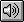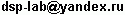MAIN PRODUCTS RESEARCH TRAINING COMPANY RUSSIAN VERSION

Robust MELP vocoder 7200 bps

Common description

Robust MELP vocoder with rate 7200 bit/s (RMELP 7200 bps) is intended for using in discrete channels with very high bit error rates (BER) up to 12-15%.

Robust MELP vocoder is built on the base of low bit rate MELP vocoder and original channel coding scheme using codes with high bit error correction capability.

Low bit rate MELP (Mixed Excitation Linear Prediction) speech coders use classical parametric model of description of speech signals. This model is implemented on the base of linear prediction filter with mixed excitation in several frequency bands.

Independent estimation of voiced/unvoiced decision in different frequency bands is providing more natural synthetic speech sounding then quality of classical LPC vocoders and near to the quality of CELP vocoders.

Implementation

RMELP 7200 bps vocoder is implemented as fixed point ANSI-C model for PC. Real-time implementation on the base of DSP TMS320C54x/C55x will be done in near future. Please ask for its parameters.

Speech samples

Here you can test speech quality of following speech samples of RMELP 7200 bps vocoder with different channel bit error rates (BER) with or without error bit grouping. (In case of modeling of error bit grouping we use 2 state Hilbert model of discrete channel with BER probability and PGB probability that mean probability of transition channel from "good" state to "bad" state and vice versa.)

 Original english speechRMELP vocoder 7200 bps, BER = 0%RMELP vocoder 7200 bps, BER = 3%RMELP vocoder 7200 bps, BER = 5%RMELP vocoder 7200 bps, BER = 7%RMELP vocoder 7200 bps, BER = 9%RMELP vocoder 7200 bps, BER = 12%RMELP vocoder 7200 bps, BER = 15%RMELP vocoder 7200 bps, BER = 5%, Pgb = 1%RMELP vocoder 7200 bps, BER = 5%, Pgb = 3%RMELP vocoder 7200 bps, BER = 7%, Pgb = 3%RMELP vocoder 7200 bps, BER = 7%, Pgb = 5%RMELP vocoder 7200 bps, BER = 9%, Pgb = 5%RMELP vocoder 7200 bps, BER = 9%, Pgb = 7%RMELP vocoder 7200 bps, BER = 12%, Pgb = 9%RMELP vocoder 7200 bps, BER = 15%, Pgb = 10%For example here you can listen the standard TETRA 7200 bps system (ACELP vocoder with standard channel coding) in the same channel conditions:

 Original english speechTETRA system 7200 bps, BER = 0%TETRA system 7200 bps, BER = 3%TETRA system 7200 bps, BER = 5%TETRA system 7200 bps, BER = 7%TETRA system 7200 bps, BER = 9%TETRA system 7200 bps, BER = 12%TETRA system 7200 bps, BER = 15%TETRA system 7200 bps, BER = 5%, Pgb = 1%TETRA system 7200 bps, BER = 5%, Pgb = 3%TETRA system 7200 bps, BER = 7%, Pgb = 3%TETRA system 7200 bps, BER = 7%, Pgb = 5%TETRA system 7200 bps, BER = 9%, Pgb = 5%TETRA system 7200 bps, BER = 9%, Pgb = 7%TETRA system 7200 bps, BER = 12%, Pgb = 9%TETRA system 7200 bps, BER = 15%, Pgb = 10%Common descriptionImplementationSpeech samples